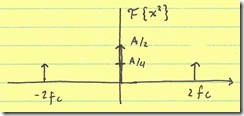# Chopping to alleviate IM2,

## IM2

Consider the fully differential amplifier shown below:$$y_1$$ is one side of the differential output and $$y_2$$ is the other. The fully differential output is $$y = y_1 – y_2$$. Without loss of generality, I have assumed infinite common-mode rejection . If we do the usual small-signal, polynomial expansion for $$y_1$$ and $$y_2$$, we get:

$$!y_1 = a_1 \times x + b_1 \times x^2$$ $$!y_2 = a_2 \times (-x) + b_2 \times (-x)^2$$ $$!y = y_1 – y_2 = (a_1 + a_2) \times x + (b_1 – b_2) \times x^2$$

This term, $$b_1 – b_2$$ basically comes down to the mismatch in the two paths. If the two sides of the amplifier match completely, there will be no IM2. So, good matching reduces IM2, but it does not completely erradicate it.

Why is this important? After all, if $$x = A \times cos(w_{c}t)$$ (i.e. a sinusoid at input frequency $$f_{c}$$:The IM2 term is $$!IM2 = A^2cos^2(w_{c}t) = \frac{A^2}{2} + \frac{A^2}{2} \times cos(2 \times w_{c}t)$$ which has terms near dc and $$2f_{c}$$, neither of which are near $$f_{c}$$:The problem is that the dc term (if the amplifier is driving a mixer) can blow right through the mixer. Any mismatch in the mixer (including duty ratio errors in its LO) will allow the dc term through and stomp on the desired baseband signal.

## Chopping

Now, consider if we insert two “chopping” mixers, each driven by a signal $$p$$, in the chain:We have $$\hat{x} = p \times x$$ and $$\hat{y} = p \times y$$. Since the chopper is a switch-mode mixer, it can only pass or negate the inputs, so $$p \in \{-1,1\}$$ and therefore $$p^2 = 1$$. Now, we have:

$$!y_1 = a_1 \times \hat{x} + b_1 \times \hat{x}^2 = a_1 \times px + b_1 \times p^{2}x^2$$ $$!y_2 = a_2 \times (-\hat{x}) + b_2 \times (-\hat{x})^2 = a_2 \times -px + b_2 \times p^{2}(-x)^2$$ $$!y = y_1 – y_2 = (a_1 + a_2) \times px + (b_1 – b_2) \times p^{2}x^{2}$$

Finally, the post-chopper multiplies $$y$$ by $$p$$ again:

$$! \hat{y} = p \times y = (a_1 + a_2) \times p^2 x + (b_1 – b_2) \times p^3 x^2$$

The $$p^2$$ term equals one:

$$! \hat{y} = (a_1 + a_2) \times x + (b_1 – b_2) \times p^3 x^2$$

The $$p^3$$ term has 3x the frequency content of $$p$$ and can generally be placed out of band.

## Caveats

One problem with the system is that the finite-bandwidth amplifier between the pre- and post- choppers adds delay to the signal. This delay needs to be tracked by the pre-mixer LO and post-mixer LO. Otherwise, the two mixers won’t align and we won’t exactly get $$p^2=1$$; instead, we’ll get $$p(t) \times p(t-\tau)$$ which gives us a little glitch (depending on how bad the delay $$\tau$$ is). This glitch yields a tone at the same frequency (and harmonics) of $$p$$. In some cases, this frequency can mix out-of-band signals back in band–which defeats the purpose of improving IM2.

Of course, there’s no reason that the $$p$$ needs to be tonal. It can, in fact, be made a spread-spectrum signal (for example, from a pseudo-noise (PN) sequence generator). In this case, the misalignment in delays will still yield bleed-through of $$p$$. However, in this case, the misalignment won’t yield a tone; it will be a spread-spectrum signal which might not be as intrusive as a single tone.

The disadvantage is that the $$p^{3}x^{2}$$ term won’t be so much shifted out of band as it will instead be spread to a wider bandwidth. Filtering will reduce some of the IM2 component, but it will not all be placed out of band.

Of course, one could combine the tonal and spread-spectrum qualities by composing $$p$$ with both a modulated and a spread-spectrum signal:

$$!p = n(t)*LO(t)$$

where $$LO(t)$$ is a pulse sequence and $$n(t)$$ is a pseudo-noise sequence. This composition has the benefit of being both spread spectrum and out-of-band (modulated to a higher frequency).

## IM3?

I’ve also been toying with the idea of whether such a system can be extended to improve IM3. I’ve concluded that it can–but not as effectively, and at the cost of some SNR. I will post the half-baked idea in the future. Perhaps someone (smarter than I) can build on the idea or will be inspired to build a better IM3 trap.

Consider a subscription via email or RSS.